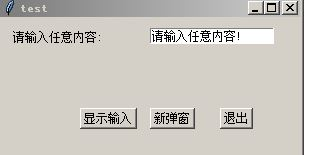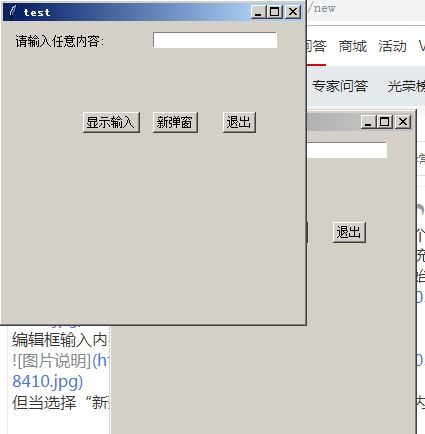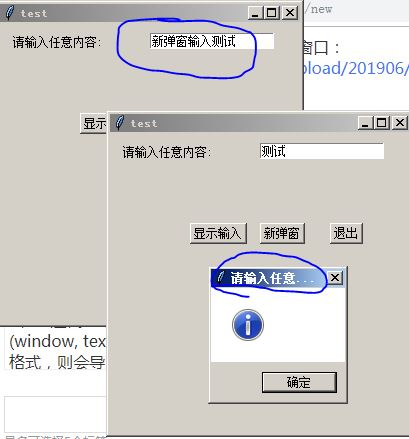Python tkinter图形界面BUTTON时间控制和编辑框返回值出现异常?

``````import tkinter as tk
from tkinter import messagebox

def newwin(title='test',geometry='300x300'):
def ShowMessage():
tk.messagebox.showinfo(input.get())
window = tk.Tk()
window.title(title)
window.geometry(geometry)
input = tk.StringVar()
input.set('请输入任意内容!')

tk.Label(window, text='请输入任意内容: ').place(x=10, y= 10)
entry_new_name = tk.Entry(window, textvariable=input)
entry_new_name.place(x=150, y=10)
btn_show = tk.Button(window, text='显示输入', command=ShowMessage)
btn_show.place(x=80, y=90)
btn_newwin = tk.Button(window, text='新弹窗', command=newwin)
btn_newwin.place(x=150, y=90)
btn_exit = tk.Button(window, text='退出', command=exit)
btn_exit.place(x=220, y=90)
return window
root = newwin()
root.mainloop()
``````1、为什么新弹窗中编辑框的显示和变化没有和变量关联起来？
2、上述代码中btn_newwin按钮定义如果改为：“btn_newwin = tk.Button(window, text='新弹窗', command=newwin)”中，如果newwin使用带实参格式，则会导致一启动就会不停新建窗口，导致递归调用过深异常出现才终止？这是为什么 ？
3、该代码存储到文件后，使用dos命令行方式执行文件无任何反应。是图形界面的程序无法在非图形界面解释器下执行吗？该怎么解决？Python tkinter图形界面BUTTON事件控制和编辑框返回值出现异常

python tkinter中button怎么绑定多个变量

tkinter想要获得控件回调函数的返回值

tkinter想要获得控件回调函数的返回值，用了global变量，但必须关闭窗口，可以不关窗口继续在窗口中使用吗？ ``` import tkinter as tk from tkinter import ttk root = tk.Tk() def Tj_go(*args): global Tj_num Tj_num = int(Tj_numberChosen.get()) return Tj_num Tj_number = tk.StringVar() Tj_numberChosen = ttk.Combobox(root, width=6, textvariable=Tj_number) Tj_numberChosen['values'] = (1, 2, 3, 4) Tj_numberChosen.grid(row=4, column=2) Tj_numberChosen.current(0) Tj_num = 1 Tj_numberChosen.bind("<<ComboboxSelected>>",Tj_go) root.mainloop() ``` 想把用户选择后的Tj_num的值返回到全局，后面还需要用

Python tkinter编程中，点击按钮怎么获取Text的内容

python tkinter里面，怎么把函数的结果按照函数原本的样式输出到text框上面？

``` import tkinter as tk import time root=tk.Tk() root.title("how to do ") root.geometry('500x600') tk.Label(root, text="显示", bg="green", font=("Arial", 12), width=5, height=1).place(x=20,y=30) def print(): EditText.insert('1.0',A()) def A(): for i in range(100): time.sleep(1) #间隔1秒输出 if i < 3: yield ("编号{}，字符串A".format(i)) if 3<i and i<5: yield ("编号{}，字符串B".format(i)) if i >5: yield ("编号{}，字符串C".format(i)) break return EditText = tk.Text(root,width=30,height=10) #创建文本框 EditText.grid(row=2,column=3) btn_test=tk.Button(root, text="按钮", command =print,width=5, height=2) btn_test.place( x=300,y=200) root.mainloop() ``` 这样子运行的结果：在text上出现：Generator Object A at 0x000002898B746CF0 如果把函数的字符串取出来： str=A() result=list(str) 这样子虽然可以输出，但是时间间隔没了、原格式也变了 期望输出结果是这样的： ![图片说明](https://img-ask.csdn.net/upload/201907/05/1562315645_427691.png) 但是如果像图片那样输出，代码可以改成： ``` def A(): for i in range(100): if i < 3: yield ("编号{}，字符串A".format(i)) if 3<i and i<5: yield ("编号{}，字符串B".format(i)) if i >5: yield ("编号{}，字符串C".format(i)) break return for i in A(): print(i) ``` 但这样子在tkinter上insert不了 请问各位大佬，有没有什么办法解决。

python tkinter里面，我怎么把函数执行的结果显示(print)到创建的Text里面？

``` import tkinter as tk root=tk.Tk() root.title("how to do ") root.geometry('500x300') tk.Label(root, text="显示", bg="green", font=("Arial", 12), width=5, height=1).place(x=20,y=30) def printtext(): EditText.insert('1.0',A()) def A(): if 2>3：print("句子1"） elif 2<0:print("句子2"） else:print("句子3") EditText = tk.Text(root,width=20,height=10) EditText.grid(row=2,column=3) btn_test=tk.Button(root, text="按钮", command =printtext,width=5, height=2) btn_test.place( x=200,y=60) root.mainloop() ``` 这样执行的结果为：tkinter.TclError: wrong # args: should be ".!text insert index chars ?tagList chars tagList ...?"

Python-Tkinter请问有什么办法可以在事件处理过程中，即时刷新Text的信息？

![图片说明](https://img-ask.csdn.net/upload/202002/01/1580541360_699863.png) 一个窗口上有四个页面，希望可以右键点击其中任意一个可以将其那一页关闭 ，试了很多次，def add_note 的第三行必须是 bind note，而不能是tab，但是改成note就变为，点击notebook任意一个地方都会删除其中一个，而不是想删哪个是哪个 原意:是想做一个类似漫画下载器一样的东西，在输入框内输入搜索的东西后，会弹出一个notebook的新的一页，之后想关闭的时候就可以将其关闭，类似选项卡一样 顺便问一下，tkinter有没有想浏览器里的选项卡一样有 ‘x’ 号 代码如下： class a: def destroy(self, event): self.note.forget(self.tab) def add_note(self, text): self.tab = Frame(self.note) self.note.add(self.tab, text=text) self.note.bind('<Button-3>', self.destroy) def main(self): root = Tk() root.geometry('500x400') self.note = Notebook(root) self.tab1 = Frame(self.note) tab2 = Frame(self.note) self.note.add(self.tab1, text='热门推荐') self.note.add(tab2, text='推荐') self.note.pack() self.add_note('123') self.add_note('123') root.mainloop() a().main()

Python3.7从Entry得到的值在get()之后返回为空

python用pymssl连接数据的时候，怎么判断是否连接成功.如果连接不成功，是否可以在窗口上显示错误信息

ArrayList源码分析(入门篇)

ArrayList源码分析 前言： 写这篇博客的主要原因是，在我上一次参加千牵科技Java实习生面试时，有被面试官问到ArrayList为什么查找的速度较快，插入和删除的速度较慢？当时我回答得不好，很大的一部分原因是因为我没有阅读过ArrayList源码，虽然最后收到Offer了，但我拒绝了，打算寒假学得再深入些再广泛些，下学期开学后再去投递其他更好的公司。为了更加深入理解ArrayList，也为

String s = new String(" a ") 到底产生几个对象？

loonggg读完需要3分钟速读仅需 1 分钟大家好，我是你们的校长。我之前讲过，这年头，只要肯动脑，肯行动，程序员凭借自己的技术，赚钱的方式还是有很多种的。仅仅靠在公司出卖自己的劳动时...

MySQL数据库面试题（2020最新版）

HashMap底层实现原理，红黑树，B+树，B树的结构原理 Spring的AOP和IOC是什么？它们常见的使用场景有哪些？Spring事务，事务的属性，传播行为，数据库隔离级别 Spring和SpringMVC，MyBatis以及SpringBoot的注解分别有哪些？SpringMVC的工作原理，SpringBoot框架的优点，MyBatis框架的优点 SpringCould组件有哪些，他们...

《经典算法案例》01-08：如何使用质数设计扫雷（Minesweeper）游戏

《Oracle Java SE编程自学与面试指南》最佳学习路线图（2020最新版）

Java岗开发3年，公司临时抽查算法，离职后这几题我记一辈子